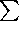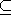## CGAL::Arr_Bezier_curve_traits_2<RatKernel,AlgKernel,NtTraits>

### Definition

The traits class Arr_Bezier_curve_traits_2<RatKernel,AlgKernel,NtTraits> is a model of the ArrangementTraits_2 concept that handles planar Bézier curves. A planar Bézier curve B is a parametric curve defined by a sequence of control points p0, ..., pn as follows:



 B(t) = (X(t), Y(t)) =k=0npk · (n!)/(k! (n-k)!) · tk (1-t)n-k .

where t[0, 1]. The degree of the curve is therefore n - namely, X(t) and Y(t) are polynomials of degree n. Bézier curves have numerous applications in computer graphics and solid modelling. They are used, for example, in free-form sketches and for defining the true-type fonts.

In our representation, we assume that the coordinates of all control points are rational numbers (namely they are given as objects of the RatKernel::Point_2 type), so both X(t) and Y(t) are polynomials with rational coefficients. The intersection points between curves are however algebraic numbers, and their exact computation is time-consuming. The traits class therefore contains a layer of geometric filtering that performs all computation in an approximate manner whenever possible, and it resorts to exact computations only when the approximate computation fails to produce an unambiguous result.

We therefore require separate representations of the control points and the intersection points. The NtTraits should be instantiated with a class that defines nested Integer, Rational and Algebraic number types and supports various operations on them, yielding certified computation results (for example, in can convert rational numbers to algebraic numbers and can compute roots of polynomials with integer coefficients). The other template parameters, RatKernel and AlgKernel should be geometric kernels templated with the NtTraits::Rational and NtTraits::Algebraic number types, repectively. It is recommended to instantiate the CORE_algebraic_number_traits class as the NtTraits parameter, with Cartesian<NtTraits::Rational> and Cartesian<NtTraits::Algebraic> instantiating the two kernel types, respectively. The number types in this case are provided by the CORE library, with its ability to exactly represent simple algebraic numbers.

#include <CGAL/Arr_Bezier_curve_traits_2.h>

### Is Model for the Concepts

ArrangementTraits_2

### Types

 Arr_Bezier_curve_traits_2::Rational the NtTraits::Rational type (and also the RatKernel::FT type). Arr_Bezier_curve_traits_2::Algebraic the NtTraits::Algebraic type (and also the AlgKernel::FT type).

### Class Arr_Bezier_curve_traits_2<RatKernel,AlgKernel,NtTraits>::Curve_2

The Curve_2 class nested within the Bézier traits class is used to represent a Bézier curve of arbitrary degree, which is defined by a sequence of rational control points. The only resriction we have is that the curve does not contain any self-intersections. In addition to the methods listed below, the I/O operators operator<< and operator>> for standard output streams are also supported. The copy constructor and assigment operator are also supported.

### Creation

Arr_Bezier_curve_traits_2<AlgKernel,NtTraits>::Curve_2 B;
default constructor.

template <class InputIterator>
Arr_Bezier_curve_traits_2<AlgKernel,NtTraits>::Curve_2 B ( InputIterator pts_begin, InputIterator pts_end);
constructs a Bézier curve as defined by the given range of control points. The value-type of InputIterator is RatKernel::Point_2.
 Precondition: The input range must contain at least two control points, and the polyline defined by these points must not be self-intersecting. That is, if we denote the control points by p0, ..., pn, then the n segements defined by pi-1pi (with 1in) must be pairwise disjoint in their interior.

### Access Functions

size_t B.number_of_control_point () returns the number of control points that define B.

typename RatKernel::Point_2 B.control_point ( size_t k) returns the kth control point. Note that the first control point equals the curve source, while the last control point equals its target. The rest of the control points do not lie on the curve.
 Precondition: k is smaller than the number of control points.

typename RatKernel::Point_2 B ( Rational t ) returns the point B(t) on the curve that corresponds to the given rational parameter value.

typename AlgKernel::Point_2 B ( Algebraic t ) returns the point B(t) on the curve that corresponds to the given algebraic parameter value.

### Class Arr_Bezier_curve_traits_2<RatKernel,AlgKernel,NtTraits>::Point_2

The Point_2 class nested within the Bézier traits class is used to represent: (i) an endpoint of a Bézier curve, (ii) a vertical tangency point of a curve, used to subdivide it into x-monotone subcurve, and (iii) an intersection point between two curves. While, points of type (i) have rational coordinates and are given as part of the input, points of the two latter types have algebraic coordinates. However, to speed up the arrangement construction, such point are not computed in an exact manner, and instead are given in an approximate representation. Note that the exact coordinates of a point may only be accessed if it is exactly computed.

In addition to the methods listed below, the copy constructor and assigment operator for Point_2 objects are also supported.

### Creation

 Arr_Bezier_curve_traits_2::Point_2 p; default constructor. Arr_Bezier_curve_traits_2::Point_2 p ( Curve_2 B, Algebraic t_0); constructs the point B(t0) on the given curve. As t0 is an algebraic number, the point has algebraic coordinates. Arr_Bezier_curve_traits_2::Point_2 p ( Curve_2 B, Rational t_0); constructs the point B(t0) on the given curve. As t0 is a rational number, the point has rational coordinates.

### Access Functions

std::pair<double, double> p.approximate () returns the approximated coordinates of p.

bool p.is_exact () returns whether the coordinates of p are computed in an exact manner.

Algebraic p.x () returns the x-coordinate of p.
 Precondition: pis exactly computed.
Algebraic p.y () returns the y-coordinate of p.
 Precondition: pis exactly computed.

bool p.is_rational () returns whether the coordinates of p are rational numbers.

typename RatKernel::Point_2 typename RatKernel::Point_2 ( p)
casts p to a point with rational coordinates.
 Precondition: p has rational coordinates.

### Class Arr_Bezier_curve_traits_2<RatKernel,AlgKernel,NtTraits>::X_monotone_curve_2

The X_monotone_curve_2 class nested within the Bézier traits is used to represent x-monotone subcurves of Bézier curves. The subcurve is defined by a supporting Bézier curve B(t) and a range of definition in the parameter space [t1, t2][0, 1], where B(t1) is the subcurve source and B(t2) is its target. Note that as the point endpoints may only be approxiamted, the parameter range definining the subcurve may only be approximately known.

It is not possible to construct x-monotone subcurves directly. Instead, use the Make_x_monotone_2 functor supplied by the traits class to subdivide a Curve_2 object into x-monotone cubcurves.

### Access Functions

 Curve_2 b.supporting_curve () returns the supporting Bézier curve of b. Point_2 b.source () returns the source point of b. Point_2 b.target () returns the target point of b. Point_2 b.left () returns the left (xy-lexicographically smaller) endpoint of b. Point_2 b.right () returns the right (xy-lexicographically smaller) endpoint of b. std::pair b.parameter_range () return the approximate parameter range defining the subcurve b.